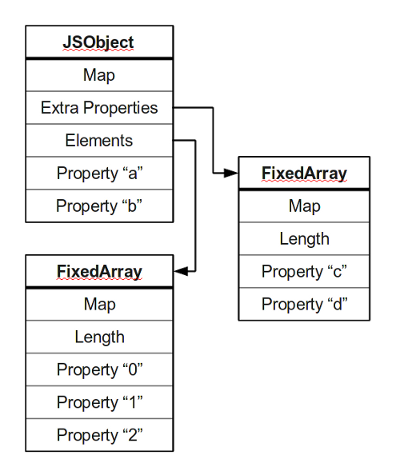## 概览## 属性的怪异属性

V8有它的难处：JavaScript标准中允许开发者以非常灵活的方式定义对象，因此很难用一种形式来高效地表示对象。一个对象基本上就是一堆属性的集合：也就是一群键值对。你可以以两种方式来访问对象的属性：

```	obj.prop
obj["prop"]
```

```	obj;    //
obj["1"];  // 这些都是同一个属性哦
obj[1.0];  //

var o = {toString: function () { return "-1.5"; } };
obj[-1.5]; // 这俩也是同一个属性
obj[o];    // 因为o转换成了字符串
```

```	var a = new Array();
a = "foo";
a.length;             // 返回101
```

## 字典模式

```	0:  map (字符串类型)
4:  length (字符数)
8:  hash code (惰性计算而来)
12: characters...
```

V8中的哈希表由一个包含键和值的大数组组成。初始时，所有的键和值都被初始化为`undefined`（一个特殊值），当有键值对插入到哈希表中时，键的哈希值被计算出来，其低位被用作数组的下标。如果数组的该位置已经被占用，则哈希表尝试（取模过后的）下一个位置，以此类推。以下是这一过程的伪代码：

```	insert(table, key, value):
table = ensureCapacity(table, length(table) + 1)
code = hash(key)
n = capacity(table)
index = code (mod n)
while getKey(table, index) is not undefined:
index += 1 (mod n)
set(table, index, key, value)

lookup(table, key):
code = hash(key)
n = capacity(table)
index = code (mod n)
k = getKey(table, index)
while k is not null or undefined
and k != key:
index += 1 (mod n)
k = getKey(table, index)
if k == key:
return getValue(table, index)
else:
return undefined
```

## 快速的对象内属性

```	function Point(x, y) {
this.x = x;
this.y = y;
}
```

V8将这种描述对象的方式称为Map。你可以假想Map为一张填满描述符的表，每一项都表示一个属性。Map也包含其他信息，比如对象的大小以及指向构造函数和原型的指针等，但这里我们主要关注这些描述符。同样结构的对象，通常会共享同一个Map。一个完成初始化的`Point`实例可能就像这样：

```	Map M2
object size: 20 (2个属性的空间)
"x": FIELD at offset 12
"y": FIELD at offset 16
```

V8处理通过一种特殊的描述符来处理这种情形：Transition。当增加一个新的属性时，除非迫不得已，我们不会创建新的Map，而是尽可能使用一个现存符合结构的Map。Transition描述符就是用来指向这些Map的。

```

Map M0
"x": TRANSITION to M1 at offset 12

this.x = x;

Map M1
"x": FIELD at offset 12
"y": TRANSITION to M2 at offset 16

this.y = y;

Map M2
"x": FIELD at offset 12
"y": FIELD at offset 16
```

```	Map M2
"x": FIELD at offset 12
"y": FIELD at offset 16
"z": TRANSITION to M3 at offset 20

this.z = z;

Map M3
"x": FIELD at offset 12
"y": FIELD at offset 16
"z": FIELD at offset 20
```

```	function Point(x, y, reverse) {
if (reverse) {
this.x = x;
this.y = y;
} else {
this.y = x;
this.x = y;
}
}
```

## 成员函数与原型

JavaScript没有类，因此它的成员函数调用与C++及Java不同。JavaScript中的成员函数只是普通的属性。在下面的例子中，`distance`只是`Point`对象的一个属性，它指向`PointDistance`函数。JavaScript中的任何函数都可以作为成员函数，并且通过`this`来访问其目标对象。

```	function Point(x, y) {
this.x = x;
this.y = y;
this.distance = PointDistance;
}

function PointDistance(p) {
var dx = this.x - p.x;
var dy = this.y - p.y;
return Math.sqrt(dx*dx, dy*dy);
}
```

C++解决这个问题的方法是虚表（译注：原文v-table）。虚表是一个存放各个虚函数指针的数组。带有虚函数的类的每个实例，都会有一个指向该类虚表的指针。当你调用虚函数时，程序会读取虚表，并按照虚表中该虚函数的地址跳转执行。在V8中，我们已经有了这么一个类似的表，它就是Map。

```

Map M0
"x": TRANSITION to M1 at offset 12

this.x = x;

Map M1
"x": FIELD at offset 12
"y": TRANSITION to M2 at offset 16

this.y = y;

Map M2
"x": FIELD at offset 12
"y": FIELD at offset 16
"distance": TRANSITION to M3

this.distance = PointDistance;

Map M3
"x": FIELD at offset 12
"y": FIELD at offset 16
"distance": CONSTENT_FUNCTION
```

JavaScript中还有另一种方法来提供公共属性，那就是通过构造函数所关联的原型对象。对于一个构造函数的实例来说，原型对象所拥有的属性，它也可以直接使用。举例来说：

```	function Point(x, y) {
this.x = x;
this.y = y;
}

Point.prototype.distance = function(p) {
var dx = this.x - p.x;
var dy = this.y - p.y;
return Math.sqrt(dx*dx, dy*dy);
}

...
var u = new Point(1, 2);
var v = new Point(3, 4);
var d = u.distance(v);
```

## 数字式属性：Fast Element

• Fast small integers
• Fast doubles
• Fast values

JavaScript不提供任何确定存储元素多少的办法。你可能会说像这样的办法，`new Array(100)`，但实际上这仅仅针对`Array`构造函数有用。如果你将值存在一个不存在的下标上，V8会重新开辟更大的内存，将原有元素复制到新内存。V8可以处理带空洞的数组，也就是只有某些下标是存有元素，而期间的下标都是空的。其内部会安插特殊的哨兵值，因此试图访问未赋值的下标，会得到`undefined`

```	// 这会大大降低大数组的性能
function copy(a) {
var b = new Array();
for (var i = a.length - 1; i >= 0; i--)
b[i] = a[i];
return b;
}
```

## 总结